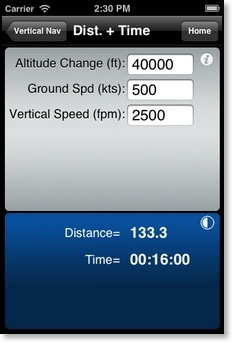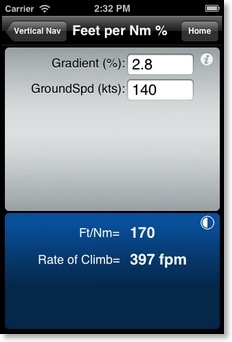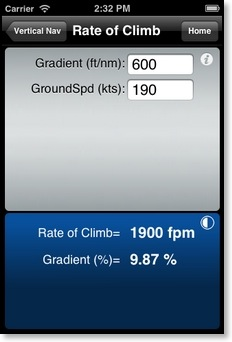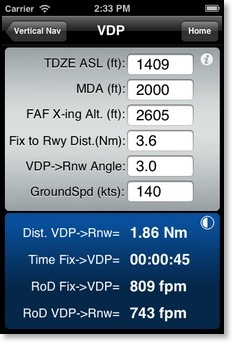FlyBy E6B
SkyWriter Software
Vertical Nav

Distance + TimeCalculates the distance and time for an altitude change. Inputs are Altitude Change, Groundspeed, and Vertical Speed in Feet per minute.

Rate of DescentCalculates the Rate of Descent and Time required for an altitude change. Inputs are Altitude Change, Groundspeed, and Distance.

Descent AngleCalculates the Rate of Descent, Time, and Distance covered in a descent. Inputs are Altitude Change, Groundspeed, and Descent angle.

Feet per NmCalculates the climb gradient in feet per nautical mile (Ft/Nm) given Vertical Speed in feet per minute and Groundspeed.

Feet per Nm (%)Calculates the climb gradient in feet per nautical mile (Ft/Nm) given Gradient in percent and Groundspeed.

Rate of ClimbCalculates the Rate of Climb (Feet per Minute) and Gradient in percent, given a required climb gradient in Feet per Nautical Mile (Req. Grad) and Groundspeed.

SCDA (Stabilized Constant Descent Angle)Used to calculate a constant descent angle FAF crossing altitude if not included on an instrument approach chart.
Note: Predicated on an assumed Threshold Crossing Height of 50 feet.
Information from this calculation is for reference use only.
Any use of a higher than published FAF crossing altitude is contingent upon prior approval by ATC.

VDP (Visual Descent Point)Used to calculate a visual descent point when not included on an instrument approach chart. Two descent rate values are calculated. The first one (Min. RoD Fix->VDP) is the minimum rate of descent required to descend from the Fix to the MDA/VDP. The second one (RoD VDP->Rnw) is the rate of descent required from the VDP to the Runway based on the flight path angle input in line 5 (VDP->Rnw Angle) and the groundspeed. Note: Information from this calculation is for reference use only.